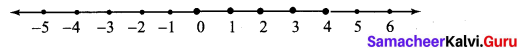Students can Download Maths Chapter 3 Algebra Additional Questions and Answers, Notes Pdf, Samacheer Kalvi 7th Maths Book Solutions Guide Pdf helps you to revise the complete Tamilnadu State Board New Syllabus and score more marks in your examinations.

## Tamilnadu Samacheer Kalvi 7th Maths Solutions Term 3 Chapter 3 Algebra Additional Questions

Exercise 3.1

Question 1.
If 4x2 + y2 = 40 and xy = b find the value of 2x + y.
Solution:
We have (a + b)2 = a2 + 2ab + b2
(2x + y)2 = (2x)2 + (2 × 2x × y) + y2 = (4x2 + y2) + 4xy = 40 + 4 × 6 = 40 + 24
(2x + y)2 = 64
(2x + y)2 = 82
2x + y = 8

Question 2.
If x2 + $$\frac{1}{x^{2}}$$ =23 find x + $$\frac { 1 }{ x }$$
Solution:
We have (a + b)2 = a2 + 2ab + b2
So (x + $$\frac { 1 }{ x }$$)2 = x2 + 2 × x × $$\frac { 1 }{ x }$$ + $$\frac{1}{x^{2}}$$
= x2 + 2 + $$\frac{1}{x^{2}}$$ = x2 + $$\frac{1}{x^{2}}$$ + 2 = 23 + 2
∵ x2 + $$\frac{1}{x^{2}}$$ = 23
(x + $$\frac { 1 }{ x }$$)2 = 25
(x + $$\frac { 1 }{ x }$$)2 = 52
x + $$\frac { 1 }{ x }$$ = 5Question 3.
Find the product of ($$\frac { 2 }{ 3 }$$ x2 + 5y2) ($$\frac { 2 }{ 3 }$$ x2 + 5y2)
Solution:
($$\frac { 2 }{ 3 }$$ x2 + 5y2) ($$\frac { 2 }{ 3 }$$ x2 + 5y2) = ($$\frac { 2 }{ 3 }$$ x2 + 5y2)2
We have (a + b)2 = a2 + 2ab + b2
Here a = $$\frac { 2 }{ 3 }$$ x2 b = 5y2
($$\frac { 2 }{ 3 }$$ x2 + 5y2)2 = ($$\frac { 2 }{ 3 }$$ x2)2 + 2 × $$\frac { 2 }{ 3 }$$ x2 × 5y2 + (5y2)2
= ($$\frac { 2 }{ 3 }$$)2 (x2)2 + $$\frac{20 x^{2} y^{2}}{3}$$ + 52 (y2)2
($$\frac { 2 }{ 3 }$$ x2 + 5y2)2 = $$\frac { 4 }{ 9 }$$ x4 + $$\frac{20 x^{2} y^{2}}{3}$$ + 25y4

Exercise 3.2

Question 1.
Solve 2x + 5 < 15 where x is a natural number and represent the solution in a number line.
Solution:
2x + 5 < 15
Subtracting 5 on both sides 2x + 5 – 5 < 15 – 5
2x < 10
Dividing by 2 on both the sides
$$\frac { 2x }{ 2 }$$ < $$\frac { 10 }{ 2 }$$
Since x is a natural number and it is less than 5, the solution is 4, 3, 2 and 1. It is shown in the number line as below.Question 2.
Solve 2c + 4 < 14, where c is a whole number.
Solution:
2c + 4 < 14
Subtracting 4 on both sides 2c + 4 – 4 < 14 – 4
2c < 10
Dividing by 2 on both the sides
$$\frac { 2c }{ 2 }$$ < $$\frac { 10 }{ 2 }$$
c < 5
Since the solutions are whole numbers which are less than r equal to 5, the solution set is 0, 1, 2, 3, 4 and 5.Question 3.
Solve -8 < -2n + 4, n is a natural number.
Solution:
-8 < – 2n + 4
Subtracting 4 on both sides
-8 -4 < -2n + 4 – 4
– 12 < – 2 n
÷ by -2, we have
$$\frac { -2n }{ -2 }$$ < $$\frac { -12 }{ -2 }$$ [ ∵ Dividing by negative number, the inequation get reversed]
n < 6
Since the solutions are natural numbers which are less then 6, we have the solution as 1, 2, 3, 4 and 5.